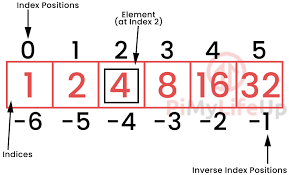Related Tags

python
array
communitycreator

# Array operations in Python

Swasthika Jain T J

## What is an array?

An array is a collection of elements of the same type. Arrays are sequence types that behave very much like lists except that the type of objects stored in them is constrained. The idea is to store multiple items of the same type together.

We can treat lists like arrays; however, we cannot constrain the type of elements stored in a list.

## Example

ar = [2, 5.5, "Hai"]
print (ar)
all elements of the array must be of the same numeric type.

Arrays in Python can be created by importing an array module.

### Syntax

Array(data_type, value_list) is used to create an array with data type and value list specified in its arguments.

import array as ar
newarr = ar.array('d', [2, 5.5, "Hai"])
print(newarr)
#you will get error
Datatype value
d Represents floating-point of size 8 bytes
b Represents signed integer of size 1 byte/td>
i Represents signed integer of size 2 bytes
I Represents unsigned integer of size 2 bytes
B Represents unsigned integer of size 1 byte
c Represents character of size 1 byte
f Represents floating-point of size 4 bytes

We need to import the array module to create arrays. For example:

import array as ar
newarr = ar.array('d', [2.1, 4.5, 5.5])
print(newarr)

## How to access array elements in Python

We can access each element of an array using the index of the element.

### Slicing Python arrays

We can access a range of items in an array by using the slicing operator:Python also has what you could call its “inverse index positions“. Using this, you can read an array in reverse.

For example, if you use the index -1, you will be interacting with the last element in the array.

Knowing this, you can easily access each element of an array by using its index number.

For instance, if we wanted to access the number 16 in our array, all we need to do is use our variable (called with square brackets, []) and the index position of the element.

### Example

The below code shows how we can access elements.

import array as ar
newarr = ar.array('d', [2.1, 4.5,3.5,4.2,3.3, 5.5])
print("First element:", newarr)
print("Second element:", newarr)
print("Last element:", newarr[-1])
print(newarr[2:5]) # 3rd to 5th
print(newarr[:])   # beginning to end


## The length of an array and how to change and add elements of an array

Use the len() method to return the length of an array (the number of elements in an array).

a = len(newarr)


### Changing element

Arrays are mutable – their elements can be changed in a similar way as lists.

import array as ar

num = ar.array('i', [1, 2, 3, 5, 7, 10])

num = 0
print(num)


We can add one item to the array using the append() method, or add several items using the extend() method.

import array as ar

num = ar.array('i', [1, 2, 3])

num.append(4)
print(num)

num.extend([5, 6, 7])
print(num)

import array as ar

num = ar.array('i', [1, 2, 3])
ln=len(num)
print(ln) #length

num = 0  #changing first element
print(num)

num.append(4) #appending 4 to array
print(num)

num.extend([5, 6, 7]) #extending numbers with 5,6,7
print(num)



## Insertion operation

Insert operation is used to insert one or more data elements into an array. Based on the requirement, a new element can be added at the beginning, end, or any given index of the built-in array.insert() function in Python.

import array as ar

num = ar.array('i', [1, 2, 3, 5, 7, 10])

num.insert(1,9)

for x in num:
print(x)

## Deletion operation in array

Deletion refers to removing an existing element from the array and re-organizing all elements of an array. The built-in remove() method is there in Python.

import array as ar

num = ar.array('i', [1, 2, 3, 5, 7, 10])

num.remove(7)

for x in num:
print(x)

## Counting elements in the array

The count() method is used to get the number of occurrences in the array.

array.count(x) returns the number of occurrences of x in the array.

RELATED TAGS

python
array
communitycreator

CONTRIBUTOR

Swasthika Jain T J
RELATED COURSES

View all Courses

Keep Exploring

Learn in-demand tech skills in half the time